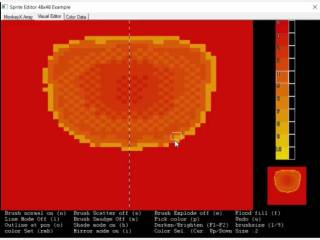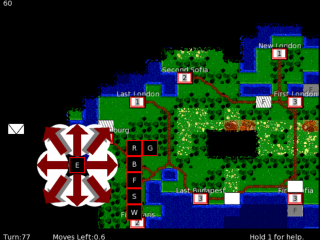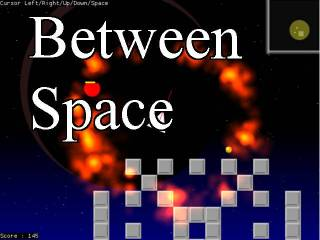-=+=- -=+=- -=+=- -=+=- -=+=- -=+=- -=+=- -=+=- -=+=- -=+=- -=+=- -=+=- -=+=- -=+=- -=+=- -=+=- -=+=- -=+=- -=+=- -=+=- -=+=- -=+=- -=+=- -=+=- -=+=- -=+=- -=+=- -=+=- -=+=- -=+=- (c) WidthPadding Industries 1987 0|538|0 -=+=- -=+=- -=+=- -=+=- -=+=- -=+=- -=+=- -=+=- -=+=- -=+=- -=+=- -=+=- -=+=- -=+=- -=+=- -=+=- -=+=- -=+=- -=+=- -=+=- -=+=- -=+=- -=+=- -=+=- -=+=- -=+=- -=+=- -=+=- -=+=- -=+=-

### Pakz

View Profile, Send Private Message
Recent Posts, Showcases, Articles, Code Snippets, Links, Wed.Workshop, Blog

### Latest Blog Entries

Pakz# Legend of Pandora and back to coding

10th October 2017

Made a part of a game I played this evening

Pakz# Normals and Dot products

23rd December 2016

Math makes no sense.

Pakz# Current Direction

22nd November 2016

Blitzmax, Monkey, Monkey2, Games

Pakz# Feedback

29th April 2016

Project now +/- 1000 lines

Pakz# Pixeljoint

28th April 2016

Quite some reference material there.

Pakz# Sprite editor getting better.

28th April 2016

New desk is getting delivered

### Latest Showcases

Pakz# Blitz Basic Sprite Editor

24th August 2018Sprite Editor

Pakz# Fire Power

16th March 2018Fire Power

Pakz# Player vs Zombies or Armed Soldiers

14th October 2017Fight in dungeons against monsters/soldiers

Pakz# A Civilization Clone v0.75

21st June 2017Browser based turn based strategy game

Pakz# Roguelike Explorer

12th April 2017Simple Html5 exploration game with Touch support

Pakz# Between Space

18th December 20162d topdown space shooter with pickups and drones